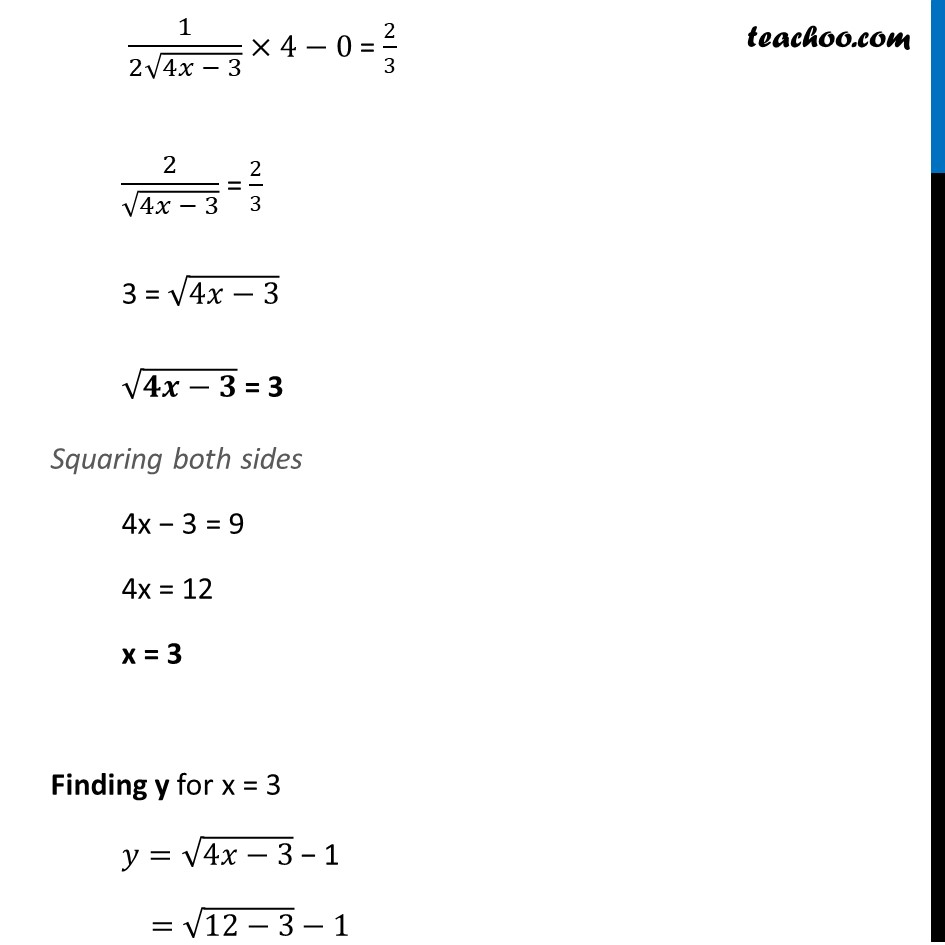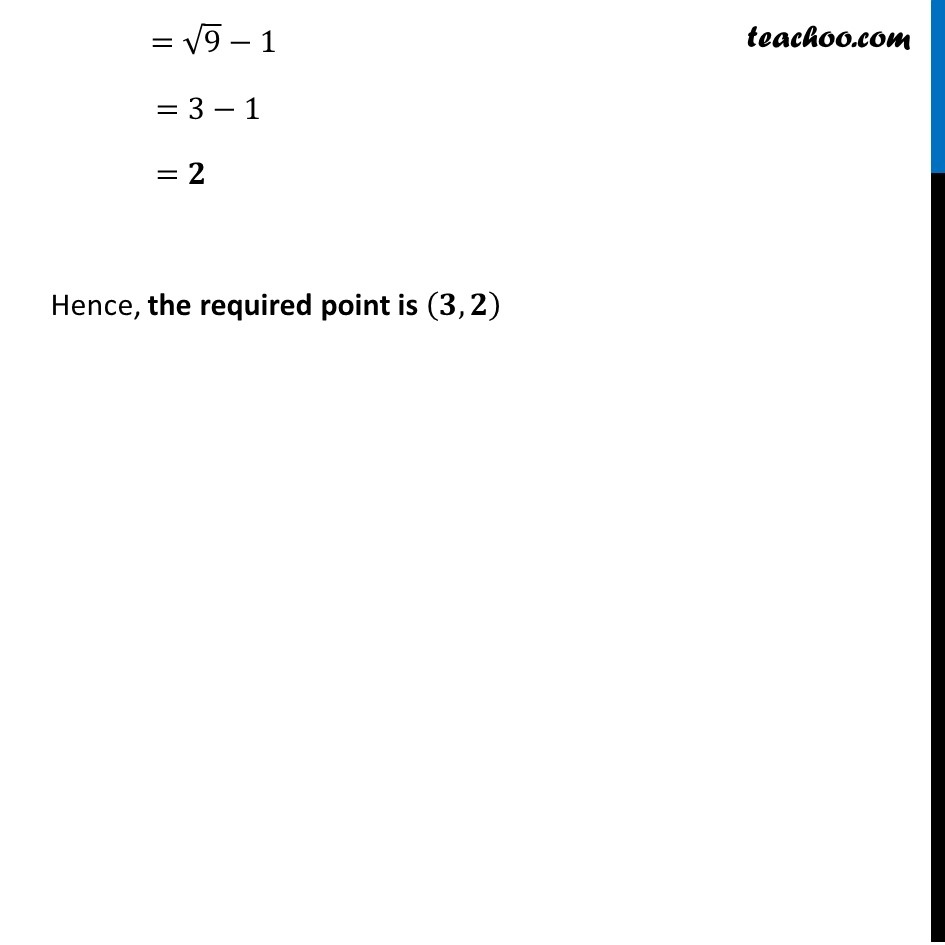Finding point when tangent is parallel/ perpendicular

Chapter 6 Class 12 Application of Derivatives (Term 1)
Concept wise### Transcript

Example 15 Find the point at which the tangent to the curve 𝑦 = √(4𝑥−3)−1 has its slope 2/3 . Given, Slope of the tangent to the curve is 2/3 We know that Slope of tangent = 𝒅𝒚/𝒅𝒙 2/3 = 𝑑𝑦/𝑑𝑥 𝑑𝑦/𝑑𝑥 = 2/3 𝑑(√(4𝑥 − 3) − 1)/𝑑𝑥 = 2/3 1/(2√(4𝑥 − 3)) ×4−0 = 2/3 2/√(4𝑥 − 3) = 2/3 3 = √(4𝑥−3) √(𝟒𝒙−𝟑) = 3 Squaring both sides 4x − 3 = 9 4x = 12 x = 3 Finding y for x = 3 𝑦=√(4𝑥−3) − 1 =√(12−3)−1 =√9−1 =3−1 =𝟐 Hence, the required point is (𝟑, 𝟐)